Themes

# What is the structural formula?

## Structural formula

In the field of chemistry , one of the most important aspects to understand is that all chemical elements have the great ability to combine with each other in different ways to produce compounds . These compounds include several molecules and these in turn have bonds which can be represented by means of a structural formula .## What is the structural formula?

The structural formula is the type of formula by means of which the locations of the chemical bonds that are located within the atoms that make up a certain molecule can be identified .

• Definition
• Characteristics of the structural formula
• What is it for
• Parties
• Structural formula types
• How it differs from a chemical formula
• Importance
• Structural formula examples

## Definition

A structural formula is a type of formula that has the ability to display a more complete picture of a compound . Some types of structural formulas can also provide a better picture of how the atoms of a given molecule are arranged in space. This type of formula is mainly used to represent molecular compounds . The complete structural formulas are those that can show all atoms those of a certain molecule, the types of bonds that join them and the way in which they are connected to each other.

## Characteristics of the structural formula

Among the main characteristics of the structural formulas, the following are mentioned:

• They are a type of graphic representation that show molecular structures .
• In it are the chemical bonds that are inside the molecules.
• You can indicate the order in which the atoms are in space.
• It has a series of symbols and short lines that are responsible for representing chemical bonds, in other words, to represent single , double , triple bonds , etc.
• The representation that is made of the atoms is made in a three-dimensional way .
• Covalent type bonds can be used in structural formulas .
• They represent the molecular structure .

## What is it for

A structural form is used to identify the location where the chemical bonds that exist between the atoms of a molecule are found, in other words, they are in charge of showing the way in which these atoms are arranged and at the same time linked. into a chemical compound. Without the structural formula, we would not know what is the spatial arrangement of the atoms in the compound. Thanks to this type of formula, chemists may be able to visualize all the changes that occur in compounds.

## Parties

The structural formulas are a kind of flat formula are composed of simple lines that handle represent a single bond between two atoms and may have two or three lines arranged in parallel by means of which is achieved represent double bonds and triples that are present. Another of its parts can be the point , which represents the unpaired electrons .

## Structural formula types

There are different ways to represent the structural formula that a compound has. Below are the different types of structural formulas.

• Dotted structure formula : This type of structural formula uses a series of electron dots to represent the electrons involved in the bond between different atoms.
• Linear bond structural formula: this linear bond structural formula is widely used and uses a series of lines and bonds that show the covalent bonds that exist between the atoms, which allows for a simpler and less cluttered presentation in the structural formula . Also, instead of drawing points, lines are drawn and each line will be the equivalent of a pair of electrons found in the bond.
• Condensed structural formula : in this type of structural formula, a series of lines between the atoms that are bonded continue to be used. It is a simpler and shorter way of being able to draw the structural formula that has the linear bond because it omits the carbon and hydrogen bonds.

## How it differs from a chemical formula

The main difference between the two is that the structural formula of a compound is a type of graphic representation that shows the molecular structure of atoms, on the other hand, the chemical formula is a representation of all the elements that are part of a certain compound. and they also show the proportion in which they are found depending on the number of atoms that are part of the molecule, in addition, the latter shows the chemical bonds and their distribution in space.

## Importance

The structural formula is important because it is responsible for being able to graphically represent the structure of the molecules in order to then be able to show how the atoms are ordered and distributed . Structural formulas are useful because they explain the properties and structure of the compound that empirical and molecular formulas cannot always represent.

## Structural formula examples

#### Structural formula of methane

Methane is an alkane-type hydrocarbon , in other words, it is a gas and its structural formula is: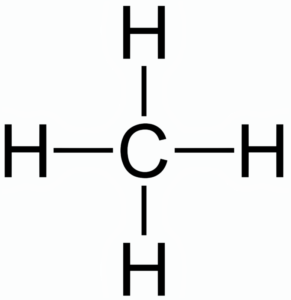#### Structural formula of water

The structural formula of water indicates the presence of two hydrogen atoms and one oxygen atom.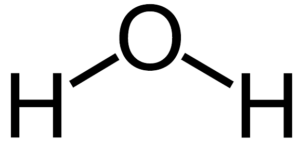#### Structural formula of benzene

The structural formula for benzene is: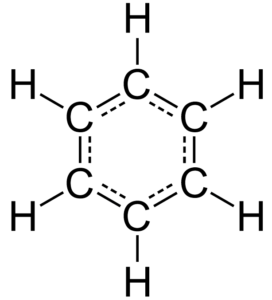#### Structural Formula of Propane

Its formula is the following: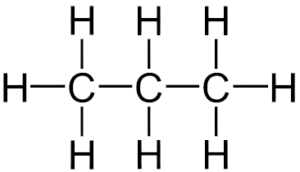#### Structural formula of ethanol

Also known by the name of ethyl alcohol, its structural formula is as follows: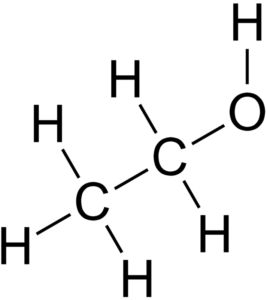#### Structural formula of starch

Its structural formula is as follows: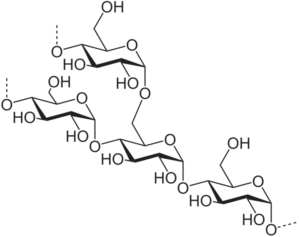#### Structural formula of heptane

Its formula is: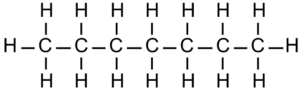#### Structural formula of phenol

The following structural formula belongs to phenol: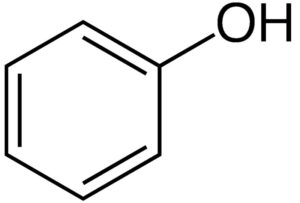#### Structural Formula of Naphthalene

Its structural formula is as follows: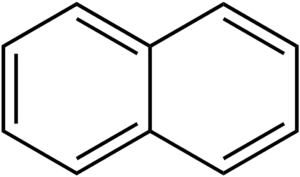Check Also
Close